Research website of Vyacheslav Gorchilin
2018-01-27
The deviation from the classical model. The second magnetic field
In this note, we show non-classical behavior of some electric circuits, comparable to the classic, and make conclusions and assumptions about the deviations. Experiments will be carried out with the help of the designer, which consists of several elements: power supply, pulse generator, switch, and transformer. The block method is interesting because at any moment of any element of this contructor can be replaced by similar, but with different parameters. For example, instead of an analog oscillator GG1 (Fig.1) you can connect digital, and instead switch CM1 — connect the other, with a bipolar transistor or a circuit with a optical isolator. It is assumed that two power supplies are already available, and concepts necessary for the rest of experiments of the elements depicted in Fig.1 and Fig.2. Another element of the designer — transformer constituting an inductor with two windings, but we'll talk about it later.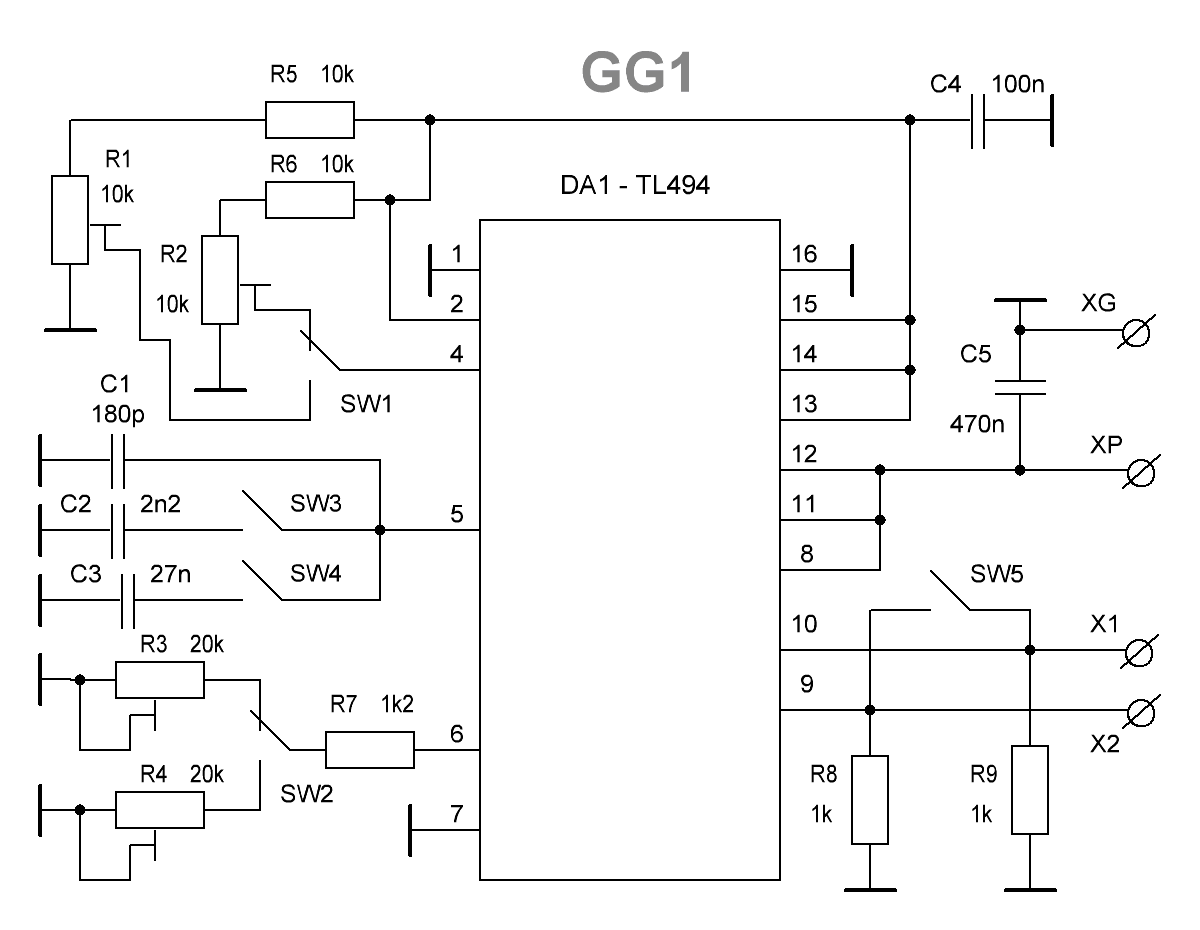Fig.1. Schematic diagram of the pulse generator GG1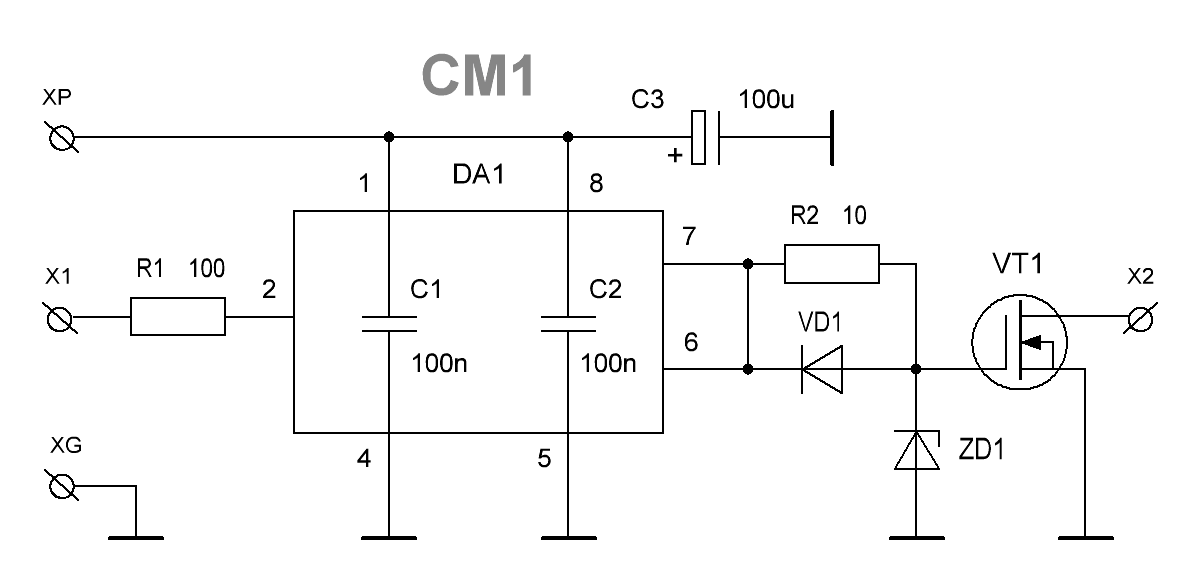Fig.2. Schematic diagram of the switch pulses CM1
Diagram of the pulse generator GG1 standard. Their outputs X1 and X2 it produces rectangular pulses with adjustable fill factor  and frequency. The fill — factor from 2 to 50%, and with the closure of key SW5 — 4 to 96%. Frequency — 2 to 600 kHz, and with the closure of key SW5 lower and upper bound of range be doubled. In the scheme added some switches: SW1 toggles coarse and fine potentiometer regulating the duty cycle using a combination of switches SW2-SW3 selects the frequency range, and SW4 switches coarse and fine potentiometer that regulates the pulse frequency. As DA1 is selected here popular chip — TL494. Potentiometer R1 and R3 — rough tuning, and R2 and R4 — mnogoobrazie. In all subsequent experiments, the switch contacts SW5 are closed.
Diagram of switch pulses CM1 is also slightly different from those stated in the datasheet. Its objective is to strengthen the impulses from GG1 to bring them in line with the input characteristics of a mosfet-transistor VT1, and to improve their fronts. Also, there are protection IC and transistor with chain R2VD1ZD1. As DA1 is applied here driver TC4420directly to the legs of the power which is soldered the capacitors C1 and C2. For VD1 can be applied to any high-speed silicon diode such as UF4007, suppressor ZD1 — 1.5KE18CA. The output switching transistor must have good characteristics of current-time transients. In experiments very well-proven mosfet 47N60.
Experience No. 1
Scheme of the experiment are shown in the following figure. It depicts: E1 — adjustable DC voltage source 6..35V, E2 — DC power supply 12V, PW1 PW2.. — power meters, OS1 — oscilloscope, C1..C2 — smoothing ceramic capacitors, VD1 — diode bridge of fast diodes, Rn — resistive load 1..3 kOhm, GG1 — generator according to Fig.1, CM1 — switch in Fig.2. Also, on the schematic there is a transformer TV1, which should be described in more detail. It is a ferrite ring with a diameter of 25..40 mm, with a wound on his 35..50 turns of double wire (bifilar), forming two identical windings. Wire diameter: 0.6..1 mm. the permeability of the core can be 1000..3000НМ. By the way, this transformer may be different, for example, made the armor core or even air option — the only difference is frequency range that the generator GG1 limited.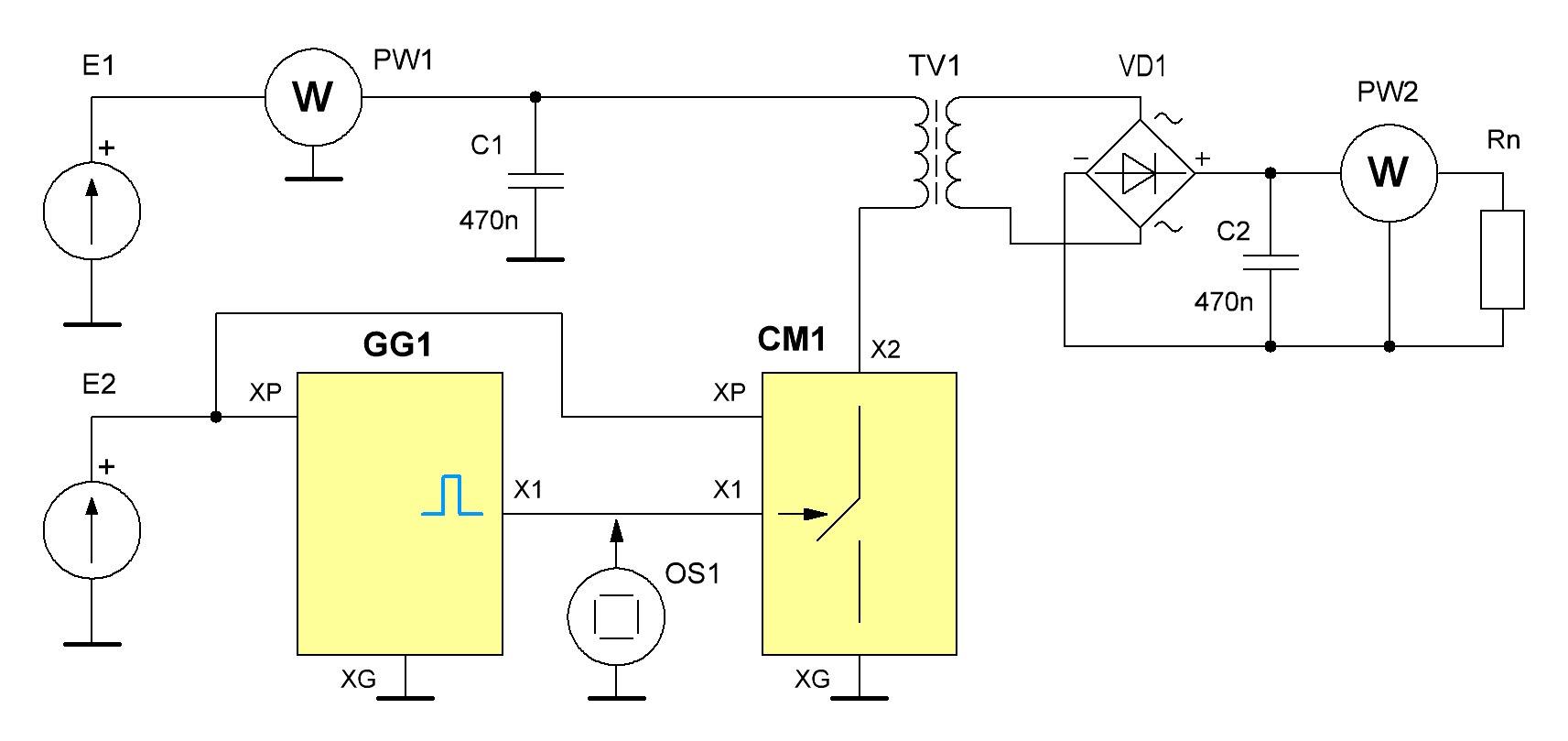Fig.3. Connection diagram of blocks for the experiment No. 1
Next, we need to introduce the coefficients of capacitance $$S$$ and a reverse — filling factor $$D$$ . The latter is more convenient and will be useful for future adjustments and schedules. On the power source E1 ustanavlivaem 10V, the second voltage E2 will always be the same — 12V — it feeds the block map. After power on stand in the generator GG1 set the fill factor to 50% and a frequency adjustment looking for max power on the load Rn. In this experiment, this maximum is of low quality and has no sharp peak. The frequency of this maximum subject as the base. Next, set the low fill factor controlling this parameter with the oscilloscope OS1. Gradually increasing the control power change at the load Rn wattmeter PW2, recording the readings in a table.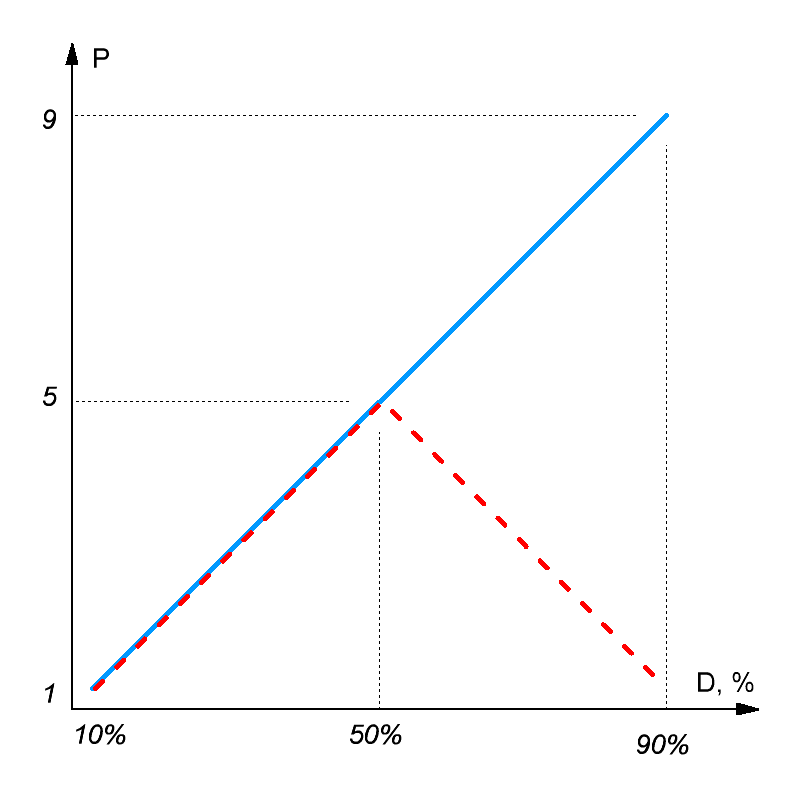Fig.4. A graph of the relative power P at the load Rn from the duty cycle D. the Red line is the classical theory, the blue — reality.
Based on the data from this table you can build a chart that will roughly correspond to Fig.4 (blue solid line).
To plot the classical model it is necessary to remember, as is the frequency spectrum of the rectangular pulse : $F(t) = A \left[D + \frac{2}{\pi} \sum_{n=1}^{\infty} \frac{1}{n} \sin(n\,\pi\,D)\cos(n\,\omega\,t) \right] \qquad (1.1)$ where: $$A$$ — amplitude rectangular pulse, $$D$$ — fill factor in relative units, $$n$$ is the number of the harmonic ($$n = 1,2,3,4,5 ...$$), $$\omega$$ — the angular frequency ($$\omega=2 \pi f$$), $$t$$ is time. The product $$A$$ and $$D$$ represents the zero harmonic or constant component, which can not be transformed to the secondary winding TV1. Then the amplitude of the remaining harmonics, which can potentially be obtained at the secondary winding of the transformer, you can search by the following formula: $a_n = \frac{2 A}{\pi\,n} \sin(n\,\pi\,D), \quad n = 1,2,3,4,5 ... \qquad (1.2)$ by Analyzing such a model it turns out that the amplitude of the first harmonic is maximum when $$D = 0.5$$, i.e., when the duty ratio is equal to 2. The amplitude of the higher harmonics will fall off symmetrically relative to this value. Thus, on the basis of the classic models and formulas (1.2) we need to obtain the schedule with the maximum power on the load when $$D = 0.5$$, as shown in Fig.4 red dotted line. In real this experience deviations from the classics start with $$D \gt 0.5$$.

In reality, because of the uneven ratio of power transmission from transformer primary to secondary, the schedule will be slightly different, but these deviations do not affect the qualitative result.

Experience No. 2
Stand for this experience is the same as for the previous (Fig. 3), but in this case we have to adjust the voltage from the source E1. Set the value of this voltage, e.g., 10V, and set the fill factor to 80%. Measure the power PW1 and PW2. Now, set the fill factor to 50%, and PW2, gradually increasing the voltage E1 will get the same power as in the previous measurement. Then measure the power PW1. It would be greater than in the first case. The author, the difference reached 15%.

In this experiment, it is possible to adjust the resonance frequency for each measurement — qualitative result is not affected.

Experience No. 3
For this experience, you will also need a source of sinusoidal low-frequency signal, which may be an industrial network of 220V. This source is included in series with the secondary winding of the transformer TV1. Here is an important initial selection of the coupling capacitor C3 is required to power on the load Rn was about the same, with independent enable network source and source from E1. While comparing coeff. fill must be set to 75%. Accurate selection of optional important only in the order of the ratio. Capacitors C3 and C4 must be rated for voltage of at least 400V.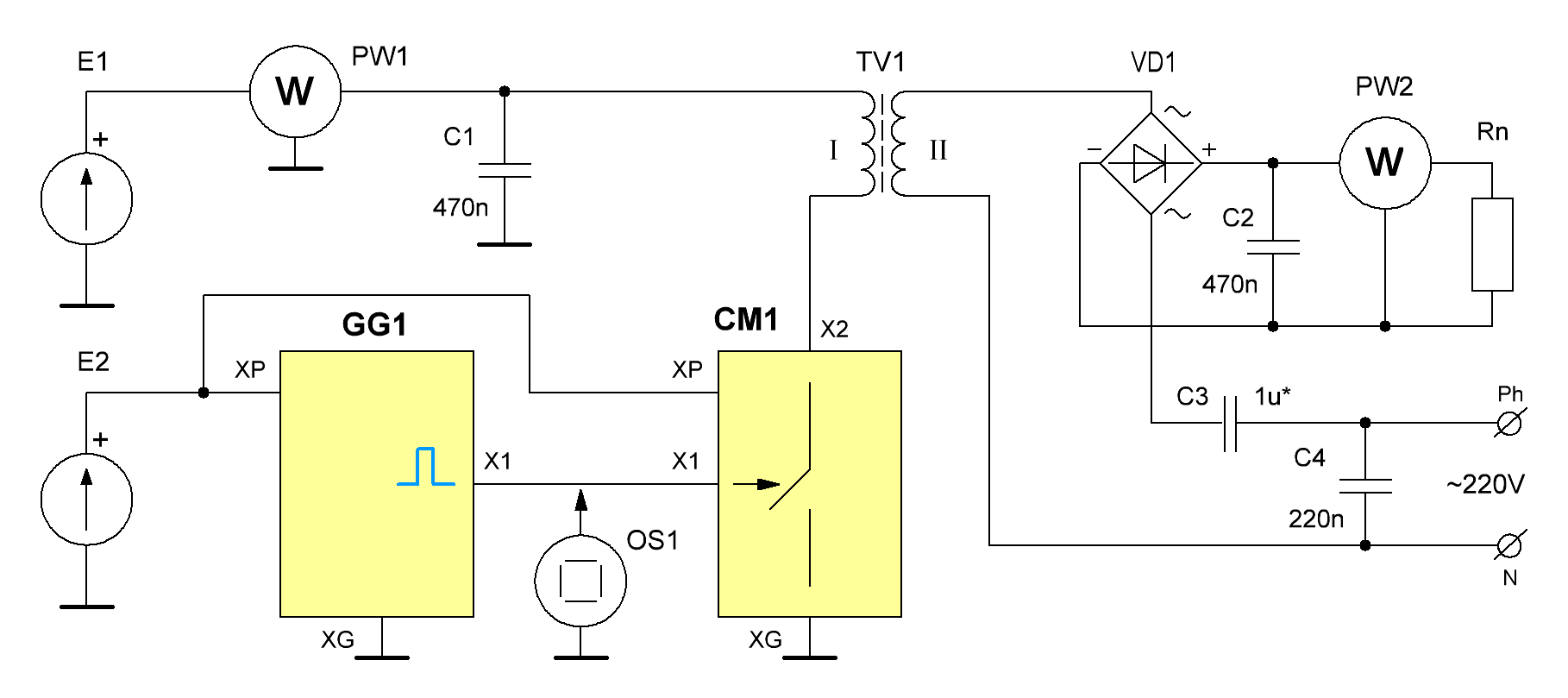Fig.5. Connection diagram of blocks for the experiment No. 3
Install coeff. fill in 85-90%, turn on all power supplies (the network including) the adjustment of frequency we find the maximum power on the load Rn, then measure the power value PW1. By the way, if close to this installation at this point will be included in the computer or oscilloscope, they can begin to restart when that power in our system is relatively small. Now install coeff. fill 50% and a frequency adjustment will adjust it for maximum power on the load Rn. In this case, adjustment of the voltage at E1 will need to pick up the same capacity on Rn, that of the first dimension. Measure the power value PW1 of this case, and it is greater than the first dimension. The author of the difference was 25-30%.

Depending on the material and core construction (or lack thereof), the number of turns of the primary and secondary windings, etc., the magnitude of the result may change in one direction or another. For example, a thicker wire it will be more than for thinner.

Conclusions and assumptions
In all three experiments we observed deviations from the classical model. The first such deviation appears due to the DC component, which in the classics can not be transformed to the secondary winding. In the second experiment — with 50% coverage should be the maximum transmission power, but in reality — at 85%. The third experience is the most difficult but also the most unusual, at least in terms of the influence fields of the transformer on the surrounding devices. Given that the q of the system is low, there should be no sharp resonant peaks, and hence the maximum power transfer yet again to be observed at 50% coverage, but in reality — at 85-90% (or more).
Apparently, in these experiments we observe another process, or rather, another unrecorded classics of the field. Nikola Tesla called it radiant, and Gennady Nikolaev — scalar or the second magnetic field . In the most General case it can have a direction vector, so we will continue to call a second magnetic field (the second MP). Analyzing these experiments the author came to the conclusion that the first and second MP are formed simultaneously with any electrical processes complementing each other. The ratio between them in the above experiments is governed by the value of the fill factor. The first MP is strictly obeys the Faraday's law  and forms in the conductor, the potential difference and the second is responsible for the emergence of additional free charges. Complementing each other they form a current.
If you change its value the second MP, apparently forms a type of electromagnetic wave (not Gertsovskiy), which can be applied through the wires (and in space), causing on the receiving end of the same action that on the transmitting — generating conductor in the additional free charges. Apparently, this is connected with the impact on electric devices in range of this field. Also, then it becomes very clear way of transmitting huge capacity for long distances in a thin conductor (single-wire energy transmission) .
Also, we recommend you to get acquainted with other experiences, which clearly shows the deviation from the classical model.

The materials used
1. The duty cycle
2. Spectra of periodic signals
3. G. V. Nikolaev. Electrodynamics of physical vacuum